﻿ Comparison of the Global Energy Cycle between Chinese Reanalysis Interim and ECMWF Reanalysis
 J. Meteor. Res.2019, Vol. 33Issue (3): 563-575PDF
http://dx.doi.org/10.1007/s13351-019-8129-7
The Chinese Meteorological Society
0

Article Information

ZHAO, Bin, Bo ZHANG, Chunxiang SHI, et al., 2019.
Comparison of the Global Energy Cycle between Chinese Reanalysis Interim and ECMWF Reanalysis. 2019.
J. Meteor. Res., 33(3): 563-575
http://dx.doi.org/10.1007/s13351-019-8129-7

Article History

Received August 13, 2018
in final form December 26, 2018
Comparison of the Global Energy Cycle between Chinese Reanalysis Interim and ECMWF Reanalysis
Bin ZHAO1,2, Bo ZHANG1, Chunxiang SHI3, Jingwei LIU3, Lipeng JIANG3
1. National Meteorological Center, China Meteorological Administration, Beijing 100081;
2. China Meteorological Administration Numerical Weather Prediction Center, Beijing 100081;
3. National Meteorological Information Center, China Meteorological Administration, Beijing 100081
ABSTRACT: The global energy cycle is a diagnostic metric widely used to gauge the quality of datasets. In this paper, the " Mixed Space–Time Domain” method for diagnosis of energy cycle is evaluated by using newly developed datasets—the Chinese Reanalysis Interim (CRAI) and ECMWF Reanalysis version 5 (ERA5), over a 7-yr period (2010–16) on seasonal and monthly timescales. The results show that the energy components calculated from the two reanalysis datasets are highly consistent; however, some components in the global energy integral from CRAI are slightly larger than those from ERA5. The main discrepancy in the energy components stems from the conversion of baroclinic process, whereas the dominant difference originates from the conversion from stationary eddy available potential energy to stationary eddy kinetic energy (CES), which is caused by systematic differences in the temperature and vertical velocity in low–mid latitudes of the Northern Hemisphere and near the Antarctic, where there exist complex terrains. Furthermore, the monthly analysis reveals that the general discrepancy in the temporal variation between the two datasets also lie mainly in the CES as well as corresponding generation and dissipation rates.
Key words: global energy cycle     transient waves     conversion terms     Chinese Reanalysis Interim (CRAI)     ECMWF Reanalysis version 5 (ERA5)
1 Introduction

Some important atmospheric systems (e.g., cyclones and anticyclones) can be identified by the kinetic energy (KE) that they possess. Gaining or losing KE can be used as a way to express the intensifying and weakening systems. Therefore, understanding the sources and sinks of KE is an effective diagnostic technique for understanding the global atmospheric circulation (Luo, 1994; Li and Zhu, 1995; Gao et al., 2006).

Lorenz (1955) proposed a global energy conversion cycle as a useful diagnostic tool to analyze the atmospheric dynamics, which has been proved to be a quantitative and crucial description for general characteristics of the atmospheric circulation. The energy conversion cycle represents the generation of available potential energy (APE) through the dissipation of KE (Dickinson, 1969), and APE as well as KE can be subdivided into mean and eddy (i.e., deviation from the mean) contributions. In this energy cycle, the mean APE is generated by solar radiation and converted into eddy APE and then eddy KE via the baroclinic instability (Stone, 1978) and zonal mean KE via barotropic processes. Conversion rates among different energies represent the horizontal and vertical momentum and sensible heat transport, and generation and dissipation rates are assessed by the corresponding energy and conversion components.

Oort (Oort, 1964; Oort and Peixóto, 1974) introduced Lorenz’s theory into the annual energy cycle and described the variability in the energy cycle at various timescales. Steinheimer et al. (2008) investigated conversions in the energy cycle for both grid-scale and subgrid-scale processes from the model parameterization schemes. The results showed that the contribution of subgrid-scale processes was much more significant than that of grid-scale processes. von Storch et al. (2012) used the NCEP simulations to estimate the energy cycle for oceans and obtained quantitative results.

The Lorenz energy cycle is formed in the spatial domain, and it can also be further introduced into a mixed temporal and spatial domain, which is named the “Mixed Space–Time Domain.” Simmons and Hoskins (1980) subdivided the eddy energy into stationary and transient waves. Stationary waves are temporally averaged and caused by diabatic and orographic forcing, and transient waves represent the departure from the temporally averaged waves, as a result of baroclinic instability (Arpe et al., 1986). Ulbrich and Speth (1991) reformatted the Lorenz energy cycle to analyze the mechanism of transient and stationary waves in the Mixed Space–Time Domain with ECMWF datasets in summer and winter. The optimized formulations better represent the details regarding the role of the interaction between dynamic and physical processes, and the results show that energy components corresponding to stationary waves are related to the planetary scale, while those which are transient correspond to synoptic-scale waves. In addition, formulation of the Mixed Space–Time Domain energy cycle is widely used in the analysis of global atmospheric energy components. Zhao and Zhang (2014) diagnosed and compared the GRAPES (Global/Regional Assimilation and Prediction Enhanced System) global model with the NCEP analysis data in July 2011. Planetary-scale processes, barotropic conversions, and baroclinic conversions were examined separately to investigate the features of each energy component's contribution. The results showed that the GRAPES model can reproduce the energy characteristics as other models do, while the main difference is caused by long-term circulation simulations.

In the meantime, a series of reanalysis datasets, such as ERA-15, ERA-40, and ERA-Interim from the ECMWF (Uppala et al., 2005; Dee et al., 2011); NCEP reanalysis 1 (R1) and NCEP–DOE R2 from NCEP and Department of Energy (Kalnay and Kanamitsu, 1996; Kistler et al., 2001; Kanamitsu et al., 2002); JRA-25 from the Japan Meteorological Agency (JMA; Onogi et al., 2005); and Modern Era Retrospective Analysis for Research and Applications (MERRA) from NASA (Rienecker et al., 2011), have been released to provide references for analysis of atmospheric circulation and variability. Some researchers have evaluated the global energy cycle by using these kinds of datasets. Li et al. (2007) re-examined the mean states of energy contributions with NCEP–DOE R2 and ERA-40 for several decades, and found that there was a general consistency between the two datasets and the near-surface processes played an important role in the conversion from mean APE to mean KE.Marques et al. (2009, 2010) compared various monthly reanalysis datasets via the energy cycle identification and found that all datasets showed similar transport trends; the obvious difference appeared in the Southern Hemisphere; and the magnitude of conversions in ERA-40 was larger than that in other datasets. Kim and Kim (2013) compared the annual and seasonal global Lorenz atmospheric energy cycles between the MERRA (NASA) and NCEP–DOE R2 datasets for over 30 years, and found that the two datasets were greatly consistent; the main difference was that the energy component magnitude in MERRA was larger.Boer and Lambert (2008) also applied the energy cycle analysis to reanalysis datasets in the Atmospheric Model Intercomparison Project 2 (AMIP2).

In 2014, the National Meteorological Information Center (NMIC) of the China Meteorological Administration (CMA) organized a Research & Development team to develop global Chinese reanalysis (CRA) datasets. The present CRA system (CRAS) was initialized by the Global System Model (GSM) v12.0.2 in Global Forecast System (GFS) of NCEP together with the Gridpoint Statistical Interpolation (GSI) v3.5 assimilation system.

In this study, the “Mixed Space–Time Domain” global energy cycle formulations are used to identify and compare the differences between the Chinese reanalysis interim (CRAI) dataset and the ECMWF Reanalysis version 5 (ERA5). The overlapping time period of 2010–16 is selected to investigate the global integration and zonal mean of global energy components. We focus on diagnosing the performance of contributions and examining the long-term temporal variations to determine the major differences and possible causes. Thereby, we can obtain more insight into the dataset performance and prepare for future applications of these reanalysis datasets.

2 Methods and data 2.1 Global energy cycle framework

Based on the work of Ulbrich and Speth (1991) (depicted in Fig. 1), all of the energy components in the global energy cycle framework can be calculated by the “Mixed Space–Time Domain” energy cycle formulations (see Appendix). The zonal APE is termed as AZ, while the zonal kinetic energy is termed as KZ. AZ is subdivided into the stationary eddy APE (ASE) and transient eddy APE (ATE), and stationary and transient eddy KE are represented by KSE and KTE, respectively. In addition to these main energy components, the conversion corresponding to the planetary-scale process CZ (conversion from AZ to KZ) shows a transient planetary wave of the temperature field interacting with the meridional wind field. The linear and nonlinear conversions for baroclinic processes [CAS (conversion from AZ to ASE), CAT (conversion from AZ to ATE), CES (conversion from ASE to KSE), CET (conversion from ATE to KTE), and CATE (conversion from ATE to ASE)] represent 1) eddy transport of sensible heat from low to high latitudes and then to KTE by vertical motion and 2) eddy transport of temperature within the latitude circle caused by the warm air rising and cold air sinking (Zhao and Zhang, 2014). Meanwhile, the conversions for barotropic processes [CKS (conversion from KZ to KSE), CKT (conversion from KZ to KTE), and CKTE (conversion from KTE to KSE)] are also important benchmarks for diagnosing the simulation of basic general circulation features such as the zonal jet stream weakening/strengthening and the transient wave interaction. Simultaneously, the generation rates of mean and eddy potential energies [GZ (generation of AZ), GSE (generation of ASE), and GTE (generation of ATE)] as well as dissipation rates of mean and eddy kinetic energies [DZ (dissipation of KZ), DSE (dissipation of KSE), and DTE (dissipation of KTE)] are calculated as the balancing terms in the equations. These terms are affected by local physical processes and integration methods, and can result in large differences.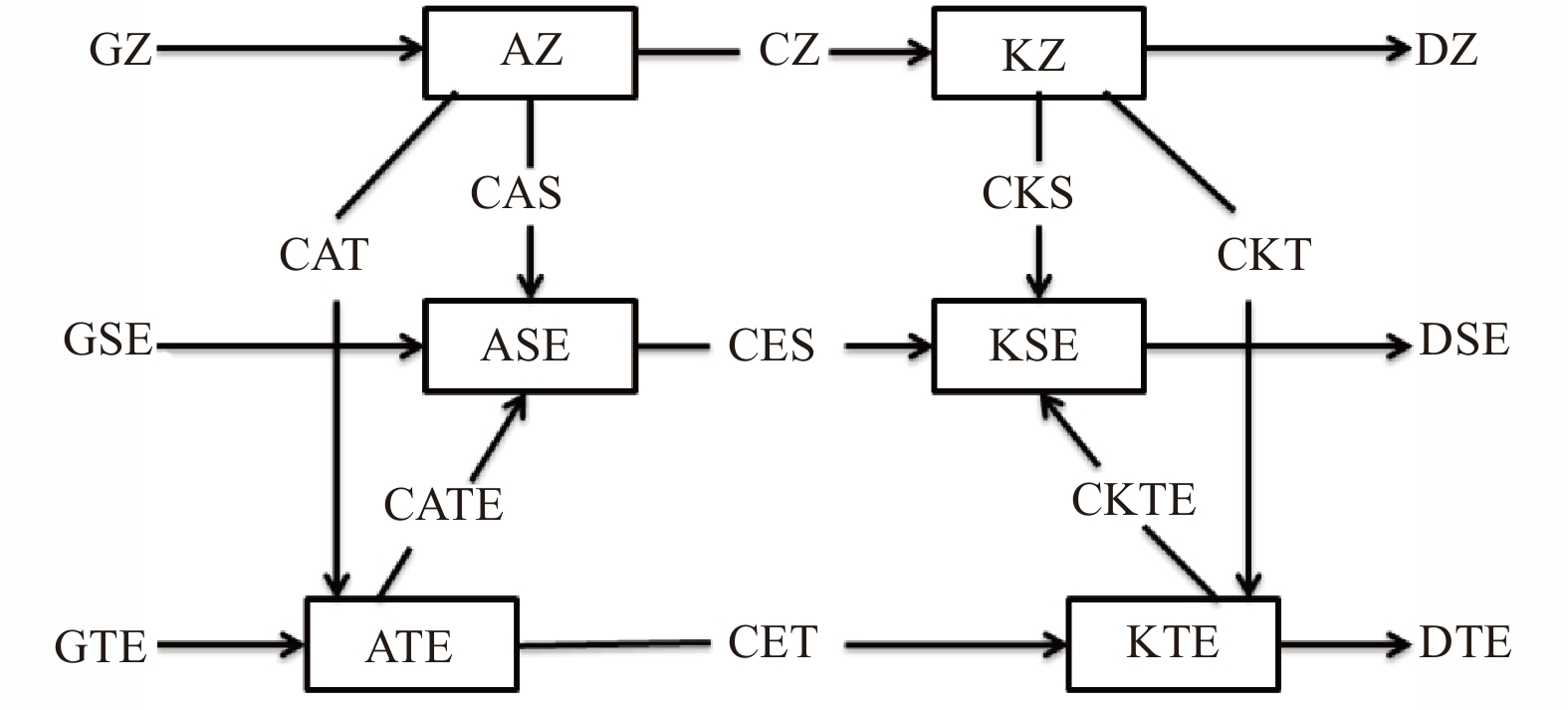Figure 1 The energy cycle framework following Ulbrich and Speth (1991). Arrows indicate the direction of energy conversion.
2.2 Data

Two reanalysis datasets, the ERA5 and CRAI, are used to calculate and compare the global energy cycle over a 7-yr period (2010–16). ERA5 is the fifth generation ECMWF global atmospheric reanalysis, which started with the First Global Atmospheric Research Program Global Experiment (FGGE) reanalysis in the 1980s, followed by ERA-15, ERA-40, and ERA-Interim. ERA5 employs the 4D-Var in CY41R2 of the Integrated Forecast System (IFS) of ECMWF, with 137 hybrid vertical levels and a top layer at approximately 0.01 hPa. All the products are interpolated to 37 pressure levels. The horizontal resolution is 31 km. This dataset provides twice-daily high-quality reanalysis products for synoptic and climate analyses.

CRAI is a 10-yr reanalysis dataset containing the standard meteorological elements from January 2007 to December 2016. It uses the GFS/GSI 3D-var system with 64 hybrid vertical levels and approximately 34-km horizontal resolution (T574). The conventional (radiosonde and surface observations) and satellite [including the reprocessed GPS Radio Occultation (GPSRO) data and Cloud Motion Wind (CMW)] data are used in the assimilation system, together with the aircraft report data for the temperature deviation correction. CRAI provides 6-hourly reanalysis products.

In this study, the overlapping 7-yr period (2010–16) 12-hourly datasets from ERA5 and CRAI are selected, and the seasonal-mean (boreal winter and summer) and monthly evolution calculated by the 12-hourly reanalysis datasets are analyzed. All products are adjusted to a horizontal resolution of 0.5°, with 17 vertical levels (1000, 975, 925, 850, 700, 600, 500, 400, 300, 250, 200, 150, 100, 70, 50, 30, 20, and 10 hPa) to ensure the compari-son consistency.

3 Results 3.1 Mean global energy cycle in January and July

Boreal winter (January) and summer (July) global mean energy components are calculated to compare the performance of CRAI and ERA5 datasets. The results are shown in Fig. 2. Note that the energy terms named with initial letters of A or K (i.e., those in the thick solid black boxes in Fig. 2) are called reservoir terms, the terms starting with letter C are called conversion terms, and those starting with letter G (D) are called generation (dissipation) terms. Fig. 2 shows that the energy reservoir terms computed from CRAI maintain high consistency with those from ERA5, in both summer and winter. As is known, AZ decomposes into a stationary wave ASE and a transient wave ATE; compared with the transient wave, the stationary wave cannot be ignored, since ASE equates to 60%–70% of the magnitude of ATE. The nonlinear conversion of CATE is an important term in the energy analysis as it directs energy from stationary to transient forms, meaning that the damping of stationary temperature via the horizontal sensible heat transient fluxes can play an important role in the general circulation (Zhao and Zhang, 2014).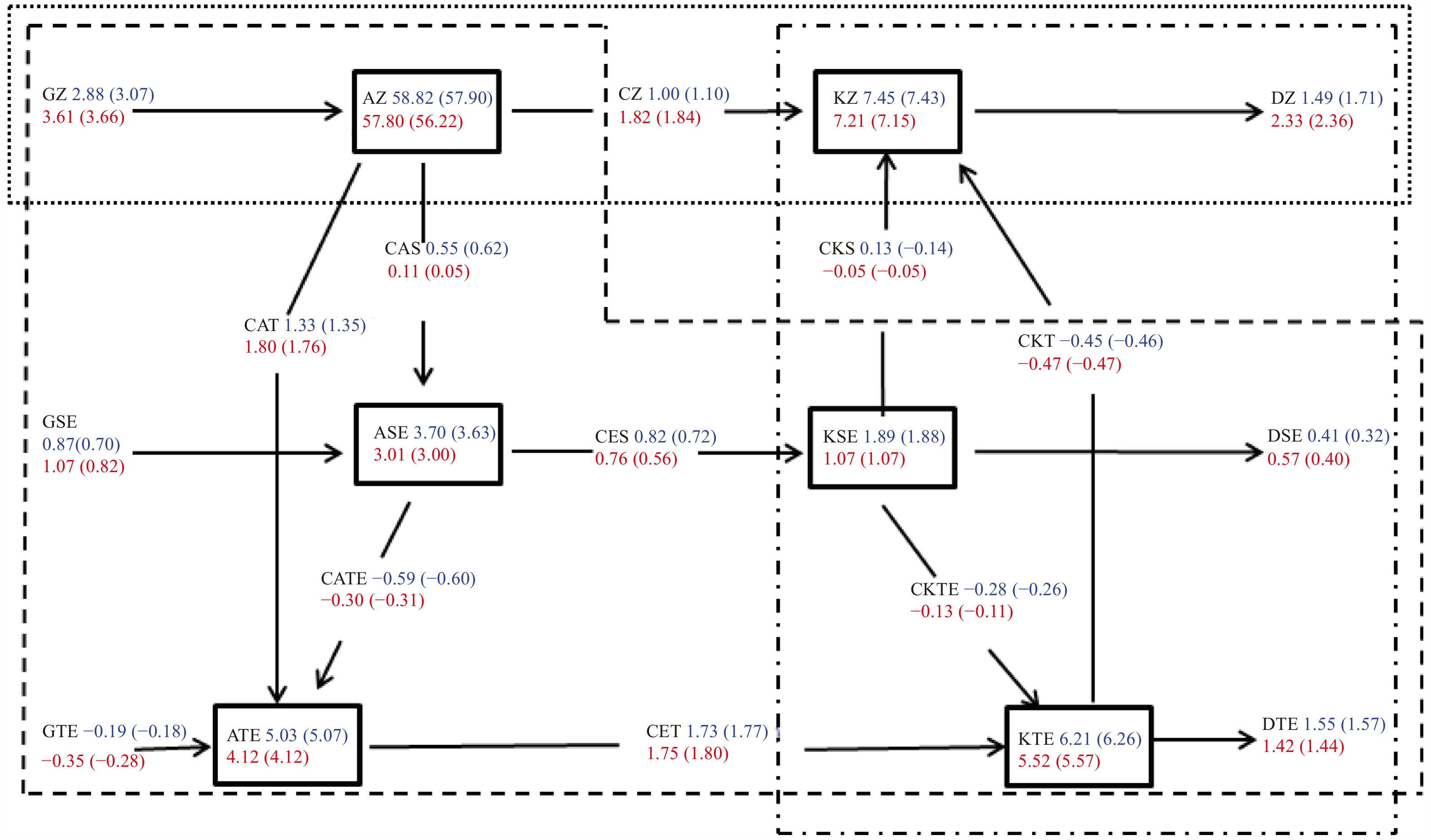Figure 2 Comparison of energy components between CRAI and ERA5 (brackets) in January (blue) and July (red). Various energy terms (boxes) and conversions are in unit of 105 J m–2 and W m–2, respectively. The dotted, dot-dashed, and dashed frames denote the planetary-scale, barotropic, and baroclinic processes, respectively.

KZ is approximate to the sum of KSE and KTE (i.e., there is almost no net global energy conversion during barotropic processes). Simultaneously, KSE is only 1/3 the magnitude of KTE, which is consistent with the previous analysis (Ulbrich and Speth, 1991).

In general, global energy components from these two reanalyses are very similar. However, the main difference comes from conversions during the baroclinic process (CES and CAS), especially in July. At the same time, we can find that generation and dissipation terms of the two reanalysis datasets show differences that are slightly larger than those in reservoir terms. In addition, we can see that almost all of the energy reservoir terms calculated by CRAI are larger than those calculated by ERA5. Meanwhile, most conversions show similar magnitude. Regarding the CZ component that is used to show the conversion between vertical wind and temperature, the descriptive ability of CRAI for Hadley cell features is relatively weaker than that of ERA5.

By comparing the globally averaged energy components, as depicted in Fig. 2, magnitude of the difference between CRAI and ERA5 in reservoir and conversion terms is larger in July than in January. Therefore, we focus on the features of energy components in summer. Figure 3 shows the mean AZ in July of 2010–16 calculated by CRAI and ERA5 as well as their differences. General patterns of energy components obtained by the two reanalysis datasets are similar, where the maximum energies are present near high latitudes in the Southern Hemisphere, while the maximum biases also exist there, suggesting that larger temperature perturbations exist in the high latitudes, and the meridional temperature gradient is larger in CRAI than in ERA5.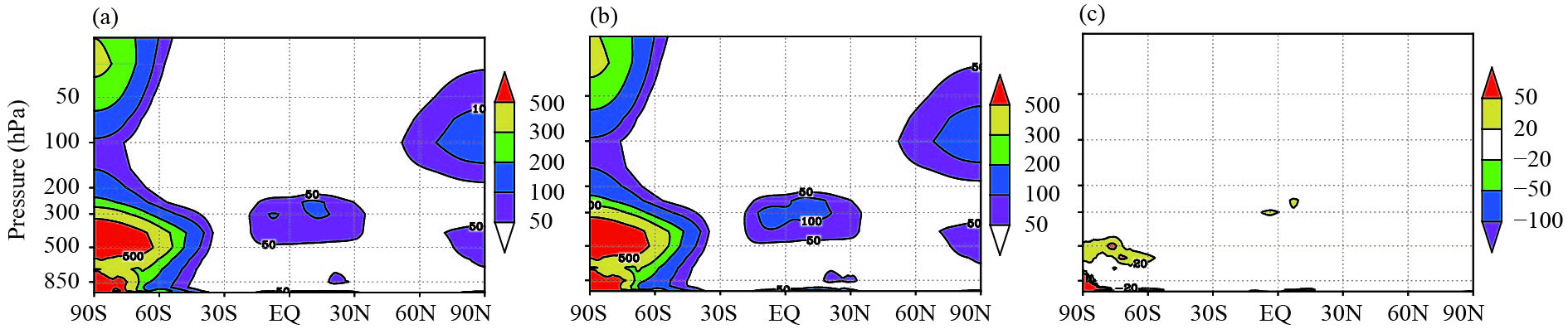Figure 3 Cross-sections of the mean AZ (J m–2 Pa–1) for July of 2010–16 from (a) ERA5, (b) CRAI, and (c) the difference between them.

Compared with AZ, the difference in KZ is much smaller (Fig. 4). Due to the existence of jet streams in the lower and upper atmosphere, the maximum KZ values computed by the two reanalysis datasets are both located over 30°S at 200 hPa. KZ of CRAI is slightly larger than that of ERA5 around 30°S, 30°N, and 60°S at the upper model levels. The difference is mainly reflected in the strength of the jet streams, but not the locations of the jet streams.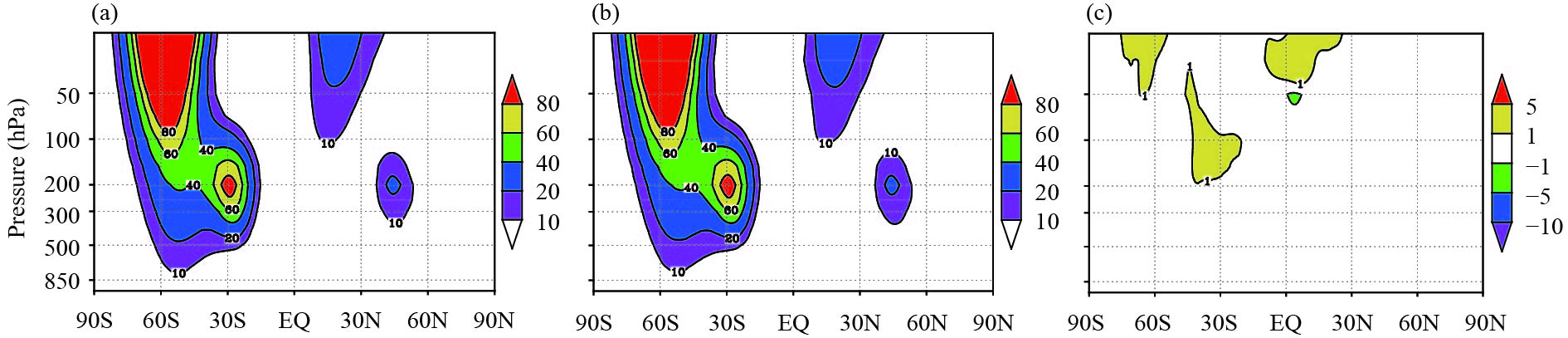Figure 4 As in Fig. 3, but for the zonal kinetic energy (KZ; J m–2 Pa–1).

For the barotropic process, the general patterns of KSE and KTE components of KZ calculated by the two reanalysis datasets show similarities in their maximum magnitudes in the Northern and Southern Hemispheres (Fig. 5). As described before, KTE is approximately three times larger than KSE. With regard to KSE, the maximum difference occurs at 150 hPa around the equator and over 0°–30°N, where the location corresponds to a large-value area of KSE in both reanalysis datasets. This is due to the presence of a stronger jet stream computed by CRAI, which strengthens the energy reservoir. The distribution of zonal mean transient wave KTE presents a different pattern compared with that of KSE. A northward maximum KTE appears in the Southern Hemisphere midlatitudes, while the maximum KTE in the Northern Hemisphere stretches southward from the north pole to low-latitude regions in the upper troposphere (300 hPa). The maximum KTE difference is observed over the tropics in the stratosphere, which reflects a weaker jet flow in CRAI.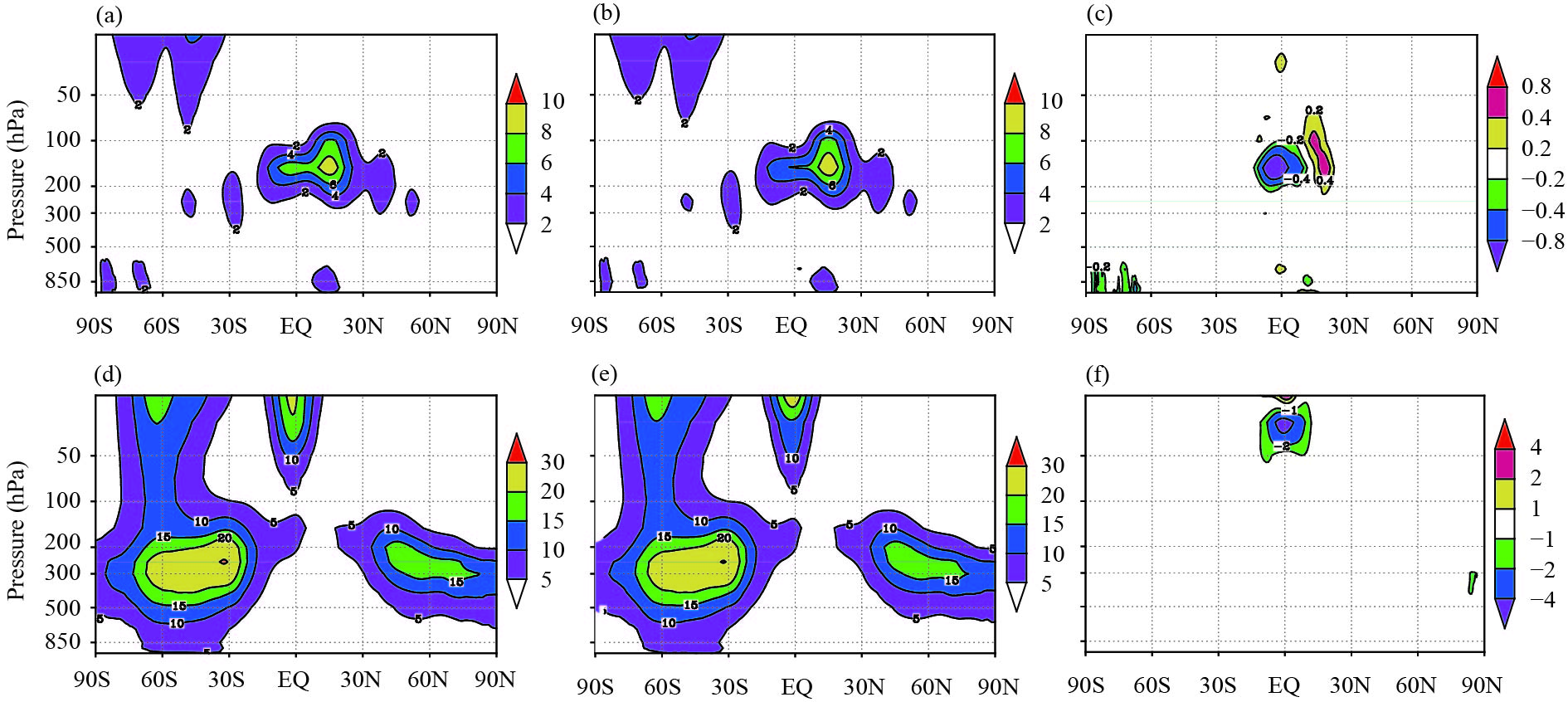Figure 5 Cross-sections of the mean (a, b, c) KSE (J m–2 Pa–1) and (d, e, f) KTE (J m–2 Pa–1) waves for July of 2010–16 from (a, d) ERA5, (b, e) CRAI, and (c, f) the difference between them.

As mentioned in Section 2.1, CKTE is the conversion term between KSE and KTE, and this nonlinear conversion directs energy from KTE to KSE (Fig. 2), which means that KE transfers from transient waves to stationary waves, resulting in strengthening of KSE. This always corresponds to a local jet maximum and plays a balancing role between the energy reservoir terms. Ulbrich and Speth (1991) proposed that the maximum CKTE is in the same region of the maximum jet stream (i.e., a positive maximum CKTE indicates a region with a local minimum KSE and maximum KTE). This phenomenon can be interpreted as a physical forcing mechanism that brings the atmosphere to a more balanced state. From the difference in the conversion terms computed by the two reanalysis datasets, we can see that a high consistency is maintained, and the main difference comes from high latitudes in the Southern Hemisphere (Fig. 6).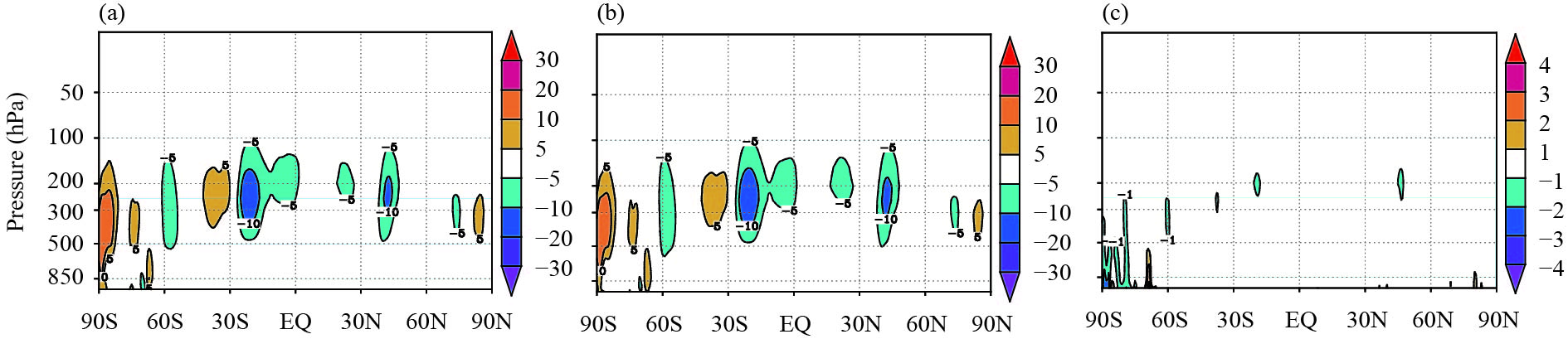Figure 6 Cross-sections of the mean conversion term CKTE (10–6 W m–2 Pa–1) for July of 2010–16 from (a) ERA5, (b) CRAI, and (c) the difference between them.

By further analyzing the detailed process associated with baroclinic conversions, the globally averaged values of stationary and transient waves maintain good consistency (Fig. 2). To comprehensively investigate the detailed performance of baroclinic conversions, the zonal mean ASE and ATE are displayed in Fig. 7. It is shown that the largest ASE values are located at 300 hPa near 30°N and over the Antarctic below 850 hPa in both CRAI and ERA5. A dominant difference in ASE also occurs in these areas. From a conceptual perspective, ASE characterizes systematic differences in temperature; it is clear that the differences between the low latitudes in the Northern Hemisphere and Antarctic region can be largely caused by the influence of complex terrain. Compared with ASE, the maximum of transient wave energy term ATE in the Northern Hemisphere is further poleward; the zonal mean ATE distribution has a structure similar to that of KTE (Figs. 5d, e), and it forms an approximately symmetrical structure in the Northern and Southern Hemispheres within the low–mid troposphere, while a weak difference is shown near the Antarctic (Figs. 7d, e).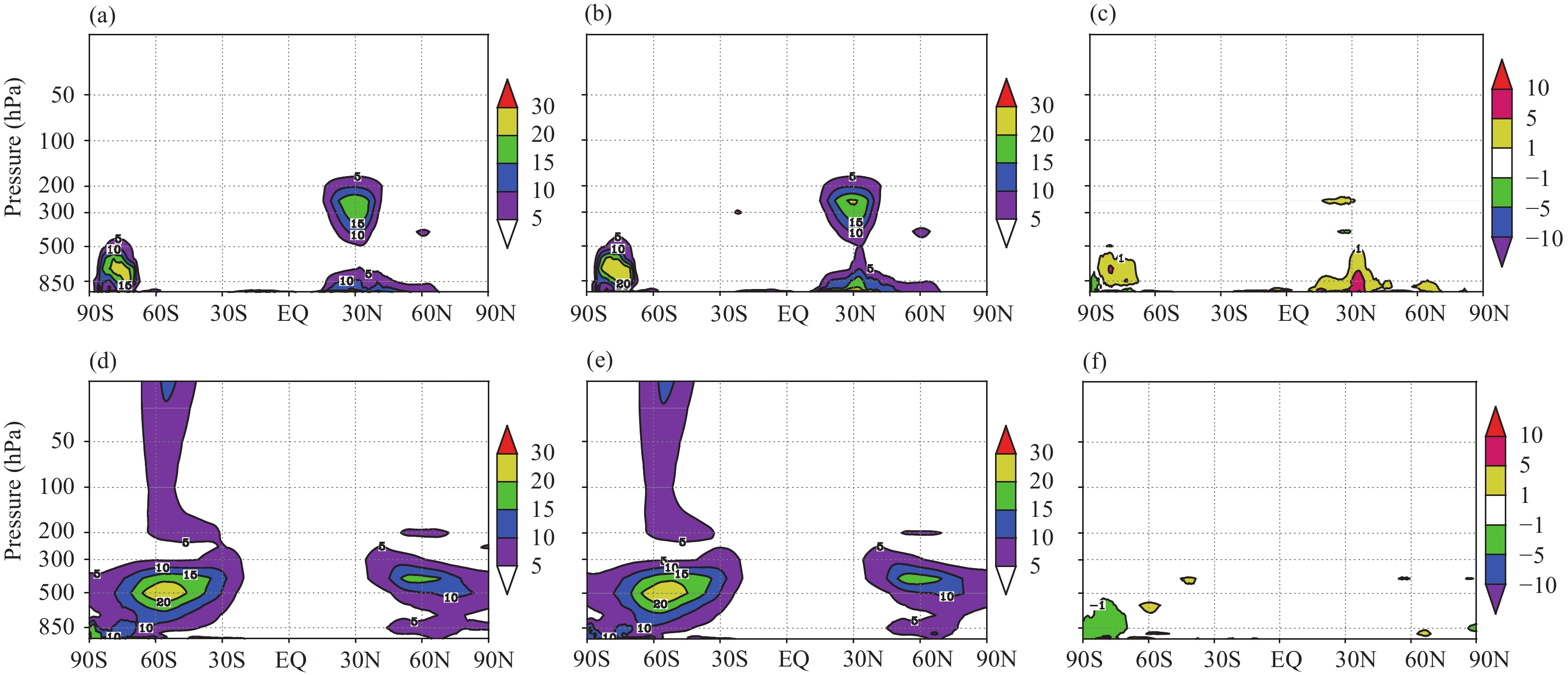Figure 7 Cross-sections of (a, b, c) ASE (J m–2 Pa–1) and (d, e, f) ATE (J m–2 Pa–1) for July of 2010–16 from (a, d) ERA5, (b, e) CRAI, and (c, f) the difference between them.

It is easy to see that from the globally averaged energy components shown in Fig. 2, the main difference in the baroclinic process comes from the conversion terms such as CES. CES can quantitatively describe the process of warm air rising and cold air sinking as a transfer process of APE to KE. The distribution characteristics of CES described by the two reanalysis datasets are consistent (Fig. 8), and the difference between the two datasets is highly similar to the case of ASE (Fig. 7c). Considering the topographical features at low–mid latitudes and near the South Pole as well as the different patterns for other energy components that have analyzed (figures omitted), it is not difficult to conclude that differences in geographic distribution of topography in the two datasets possibly dominates the differences in the distribution of CES.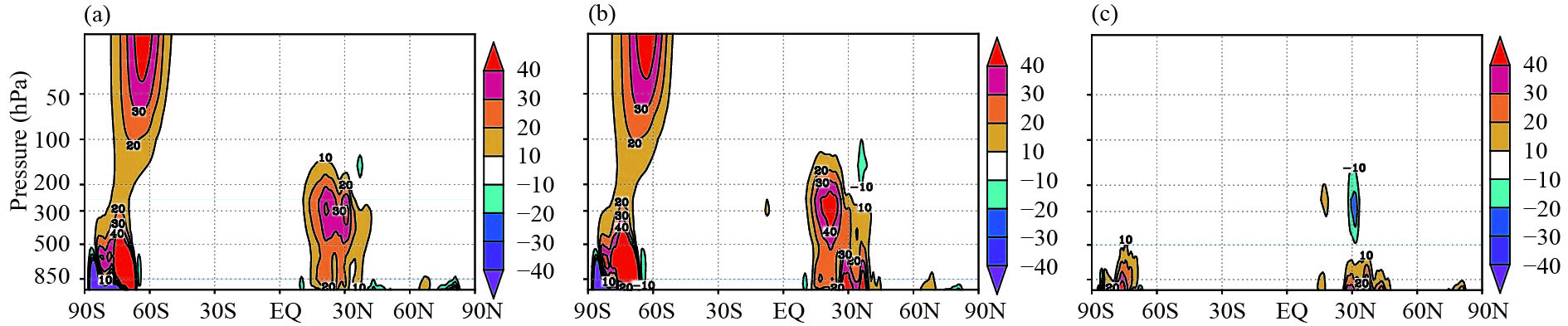Figure 8 Cross-sections of the mean conversion term CES (10–6 W m–2 Pa–1) for July of 2010–16 from (a) ERA5, (b) CRAI, and (c) the difference between them.

To identify the sources for the difference (bias) in energy components between the two reanalysis dataset, we examine the performance of each forecast variable separately (Fig. 9). The bias in wind field is concentrated near the equator; where zonal wind bias is located in the upper layer (similar to KTE) while meridional wind bias occurs from the surface to upper levels. Meanwhile, there is certain bias near the Antarctic. The temperature bias is mainly concentrated in the lower troposphere, where there is an obviously negative bias at high latitudes in the Southern Hemisphere, while the largest positive deviation appears in the Northern Hemisphere near 30°N, and the overall distribution is similar to that of ASE (Fig. 7c). In terms of specific humidity, we can see that CRAI represents a certain dry pattern at low–mid latitudes in the Northern Hemisphere compared to ERA5; the large difference is mostly concentrated near 30°N, which is simi-lar to that for temperature. For the vertical velocity field, the ascending motion in CRAI near the equator is obviously stronger than that in ERA5. In contrast, low latitudes in the Northern Hemisphere correspondingly show strong sinking motion. Near 30°N, the low-level sinking motion is slightly larger, while the mid–upper troposphere is dominated by strong upward air motion. At the same time, it can be found that the vertical velocity bias is concentrated in the Northern Hemisphere, while only some excessive sinking motion occurs in the lower layer at high latitudes of the Southern Hemisphere.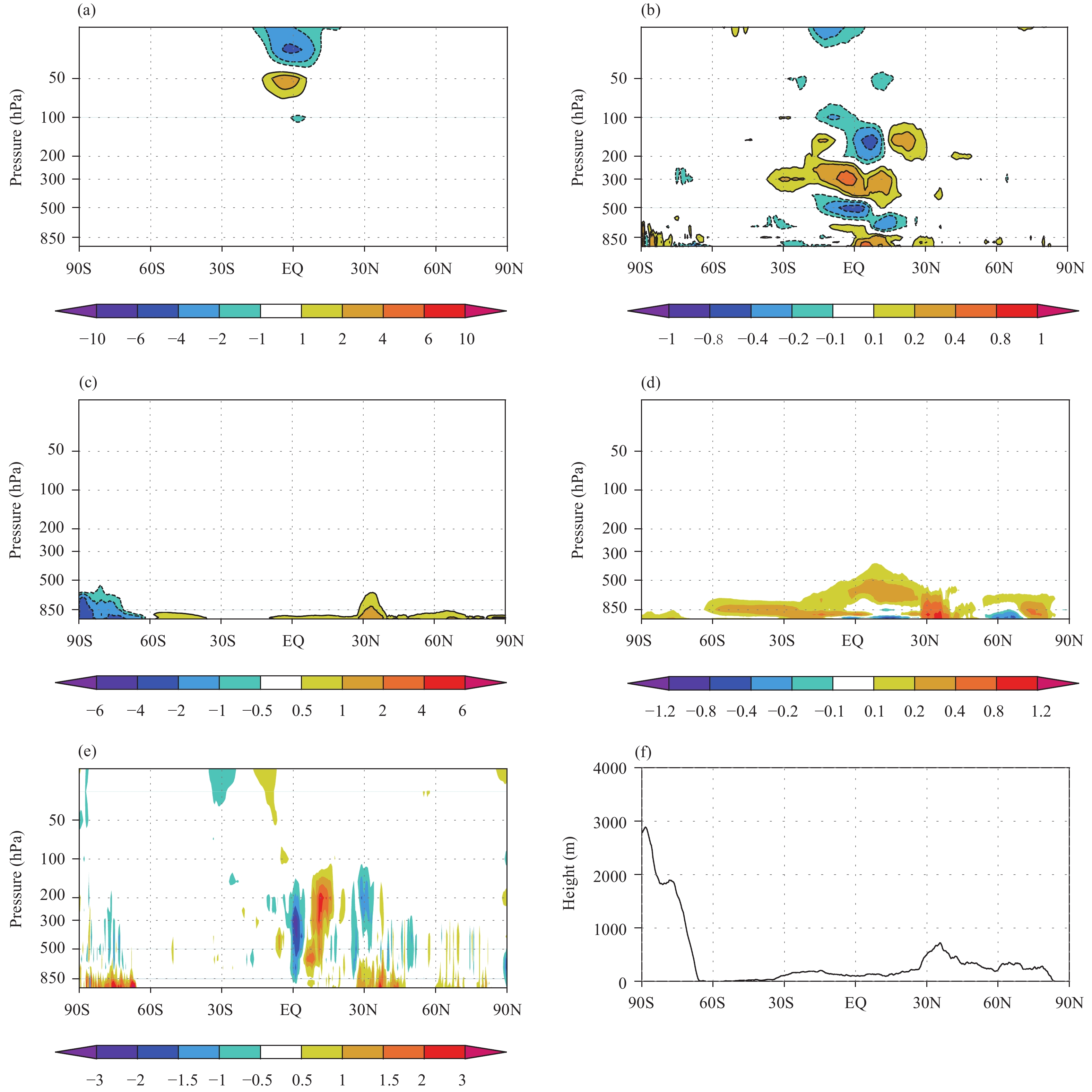Figure 9 The zonal mean difference between ERA5 and CRAI for (a) zonal wind (m s–1), (b) meridional wind (m s–1), (c) temperature (K), (d) specific humidity (g kg–1), and (e) vertical speed (omega; Pa s–1) for July of 2010–16. Panel (f) shows the topography of CRAI.

CES is calculated by using the formula A11 in Appendix. In A11, ω represents the vertical p-velocity, Tv represents the virtual temperature, R represents the gas constant, p represents the pressure, g represents the gravity of earth, and * represents the deviation from zonal mean. Apparently, CES is related to the vertical p-velocity and virtual temperature, and the latter can be calculated with the temperature and specific humidity. Therefore, by comparing the p-velocity, temperature, and humidity, it is found that there is clearly a reason for the large difference in the energy components at midlatitudes and near the Antarctic. According to these common features, it is not difficult to find that the large differences should be related to the complex terrains at the corresponding locations (Fig. 9f).

3.2 Monthly evolution

In the above section, the mean state of global energy cycle is investigated. Here, the long-term monthly mean characteristics of energy reservoir and conversion terms are summarized, together with the corresponding correlation between ERA5 and CRAI during 2010–16 (Table 1). In Table 1, the monthly mean values are calculated by averaging the global energy components over the 7-yr period, and confidence intervals with a 99% confidence level are used to estimate the distribution range. It is shown that the energy reservoir and conversion terms are mainly within this significant interval. To further illustrate the monthly evolution of energy reservoirs and conversions, standard deviation is calculated (Table 1) to represent the dispersion characteristics. Table 1 clearly shows that energy reservoirs computed by the two reanalysis datasets maintain an obvious consistency, with some correlation coefficients of above 99%. The spreading of AZ calculated by CRAI is higher than that by ERA5; however, the subdivided components (ASE and ATE) are basically similar.

Table 1 Monthly mean values and corresponding correlations of the globally averaged energy components for ERA5 and CRAI in 2010–16
 Component ERA5 CRAI Correlation Mean Standard deviation Mean Standard deviation AZ (105 J m–2) 56.75 ± 0.55 2.56 57.82 ± 0.56 2.62 0.992 KZ (105 J m–2) 7.29 ± 0.13 0.61 7.22 ± 0.11 0.50 0.999 ASE (105 J m–2) 3.15 ± 0.12 0.56 3.34 ± 0.12 0.56 0.993 KSE (105 J m–2) 1.77 ± 0.10 0.46 1.77 ± 0.10 0.46 0.999 ATE (105 J m–2) 4.15 ± 0.06 0.30 4.11 ± 0.06 0.29 0.997 KTE (105 J m–2) 5.16 ± 0.05 0.24 5.11 ± 0.05 0.24 0.998 CZ (W m–2) 1.10 ± 0.06 0.28 1.17 ± 0.06 0.28 0.942 CAS (W m–2) 0.42 ± 0.04 0.20 0.36 ± 0.04 0.20 0.989 CKS (W m–2) –0.15 ± 0.01 0.07 –0.14 ± 0.01 0.06 0.993 CAT (W m–2) 1.62 ± 0.04 0.18 1.62 ± 0.04 0.19 0.986 CKT (W m–2) –0.45 ± 0.01 0.07 –0.45 ± 0.01 0.06 0.994 CES (W m–2) 0.54 ± 0.03 0.14 0.71 ± 0.03 0.13 0.915 CET (W m–2) 1.76 ± 0.02 0.11 1.74 ± 0.02 0.10 0.985 CATE (W m–2) –0.41 ± 0.02 0.10 –0.39 ± 0.02 0.11 0.993 CKTE (W m–2) –0.04 ± 0.01 0.06 –0.06 ± 0.01 0.07 0.995 GZ (W m–2) 3.14 ± 0.06 0.28 3.15 ± 0.07 0.32 0.916 GSE (W m–2) 0.53 ± 0.04 0.18 0.74 ± 0.04 0.21 0.976 GTE (W m–2) –0.27 ± 0.02 0.09 –0.27 ± 0.02 0.09 0.907 DZ (W m–2) 1.70 ± 0.05 0.23 1.76 ± 0.05 0.24 0.914 DSE (W m–2) 0.36 ± 0.02 0.10 0.52 ± 0.02 0.10 0.822 DTE (W m–2) 1.34 ± 0.01 0.06 1.35 ± 0.01 0.07 0.957

On the contrary, the standard deviation of KZ in CRAI is smaller than that in ERA5, while the subdivided terms (KSE and KTE) maintain a high level of agreement. The main difference lies in the conversion terms, where the correlation coefficient for CES is only 91.5%, while that for CZ is 94.2%. There is no obvious difference in the spreading characteristics (standard deviation). It seems difficult to find the main source of difference between the two reanalysis datasets. The large difference in the monthly mean values of CES (0.54 W m–2 for ERA5 and 0.71 W m–2 for CRAI) leads to subsequent evolution difference. The globally averaged energy generation and dissipation terms are based on energy reservoirs and conversions by budget equations. Obviously, in the equations with large differences in conversion terms, the correlation between generation and dissipation terms is weak. Therefore, temporal differences in energy reservoir and conversion terms are not very important in diagnosing the generation and dissipation rates.

In regard to the annual cycle of global energy reservoir terms, Fig. 10 displays the annual evolution of these energy components averaged in 2010–16. It is clear that these energy terms shows apparent periodic variation characteristics. Compared to APE, KE terms are more consistent with each other. Magnitudes of AZ and ASE in CRAI are obviously higher than those in ERA5, and the largest difference appears in summer (2.7% larger than ERA5 for AZ in August and 10.1% larger than ERA5 for ASE in July). However, the evolution characteristics are similar between CRAI and ERA5.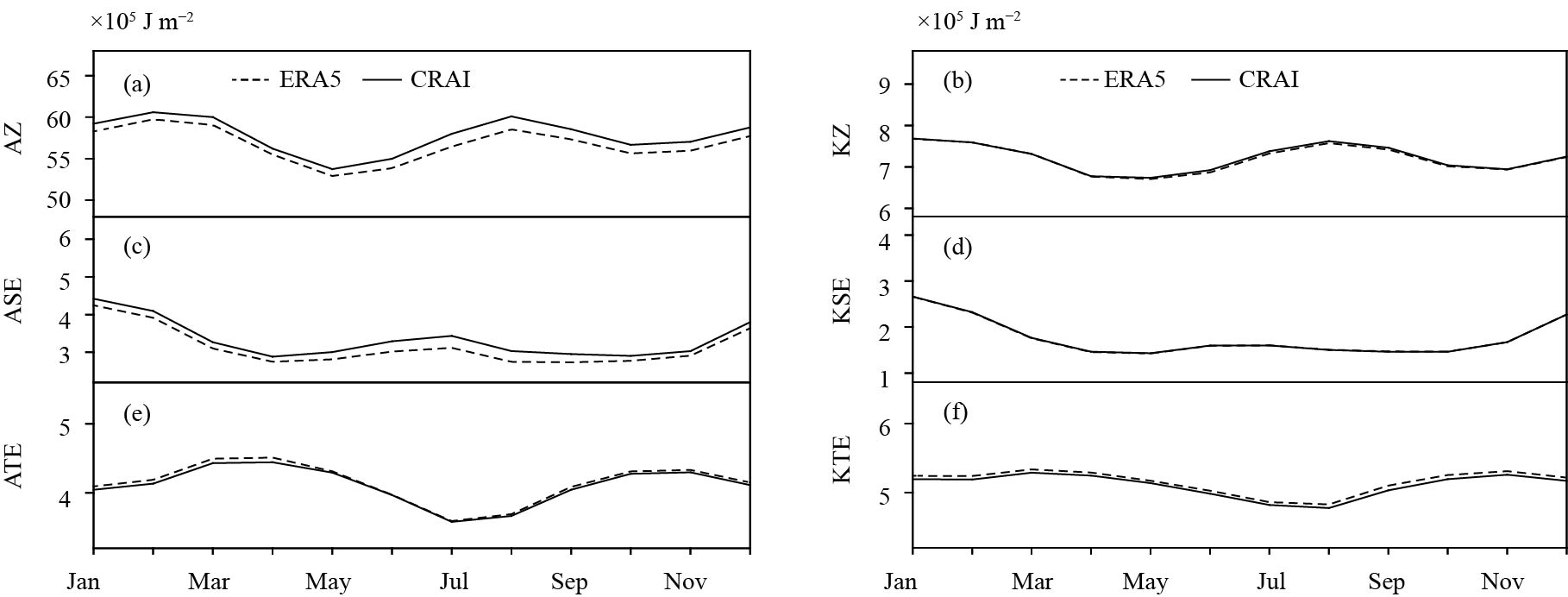Figure 10 Monthly mean (2010–16) time series of the globally averaged (a) AZ, (b) KZ, (c) ASE, (d) KSE, (e) ATE, and (f) KTE from ERA5 (dashed line) and CRAI (solid line). The unit of each variable is 105 J m–2.

Different from energy reservoir terms, energy conversion terms display some discrepancies, especially for conversions from ASE to KSE (CES; Fig. 11). Clearly, CZ and CET maintain relatively similar evolution features, while CES, which represents the conversion from ASE to KSE, presents certain differences. The variation between boreal spring and summer is obviously larger than that between boreal autumn and winter. The largest CES difference appears in July and CRAI is about 37.8% larger than ERA5. As previously analyzed, the eddy conversion term from APE to KE is essentially caused by variations in the temperature and vertical velocity in the latitudinal circulation. Therefore, positive biases in the temperature and vertical velocity at 30°N and near the surface over the Antarctic play an important role in this corresponding difference.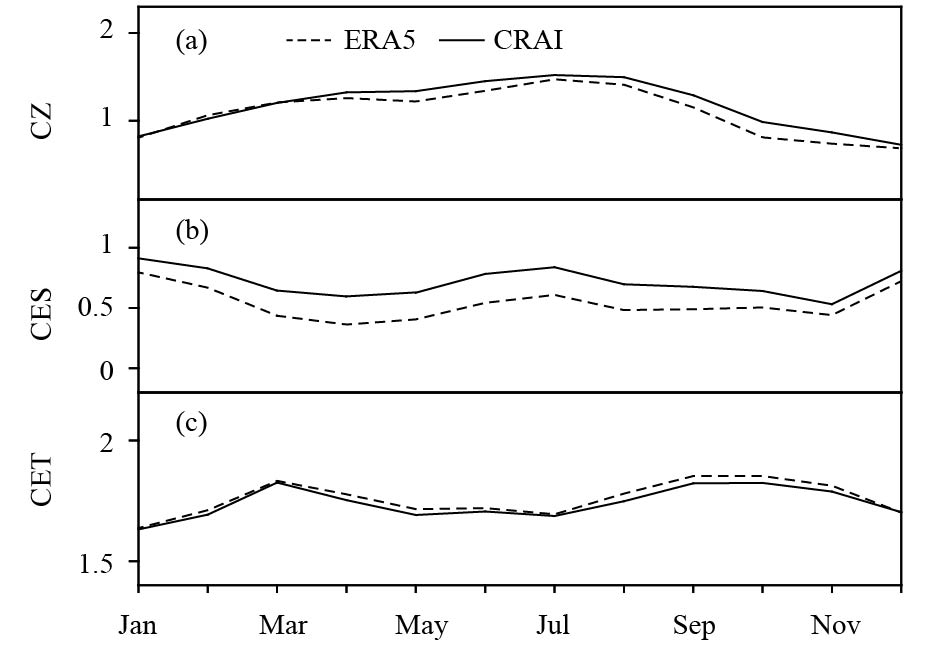Figure 11 Monthly mean (2010–16) time series of the globally averaged (a) CZ, (b) CES, and (c) CET from ERA5 (dashed line) and CRAI (solid line). The unit of each variable is W m–2.

By further analyzing the other conversion terms in baroclinic and barotropic processes (Fig. 12), it is inferred that conversion terms between the mean and eddy potential energy are produced by the eddy transport of heat and temperature gradient. Moreover, the eddy transport of momentum and gradient in the mean angular rotation creates conversion rates between the mean and eddy KE. It can be concluded that both the KE and potential energy conversion terms present high degrees of consistency based on the two reanalysis datasets, and CRAI products can effectively depict seasonal evolution characteristics of the temporal evolution in various energy components.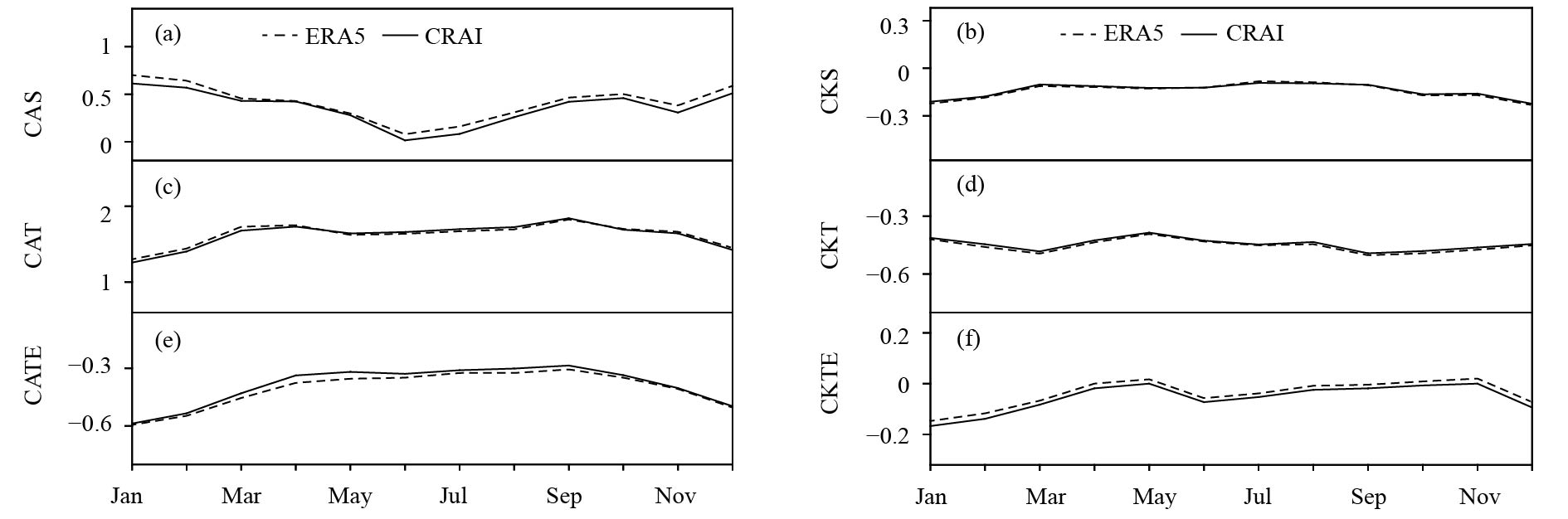Figure 12 Monthly mean (2010–16) time series of the globally averaged (a) CAS, (b) CKS, (c) CAT, (d) CKT, (e) CATE, and (f) CKTE from ERA5 (dashed line) and CRAI (solid line). The unit of each variable is W m–2.

The generation of potential energy via the radiative heating and the dissipation of KE via the friction can be barely measured directly. They can only be calculated by the balance of energy reservoir terms. Figure 13 shows the annual evolution of globally averaged generation and dissipation terms based on CRAI and ERA5. Obviously, the largest difference comes from the generation and dissipation of stationary waves (i.e., the systematic difference of reanalysis datasets); in terms of GSE, the largest difference exists in summer and the CRAI value is larger than the ERA5 value by about 34.7% in June. For the planetary-scale and transient waves, the two reanalysis datasets maintain a high degree of consistency, which are now given more attention in climatological research. The CRAI dataset seems to have a good application prospect.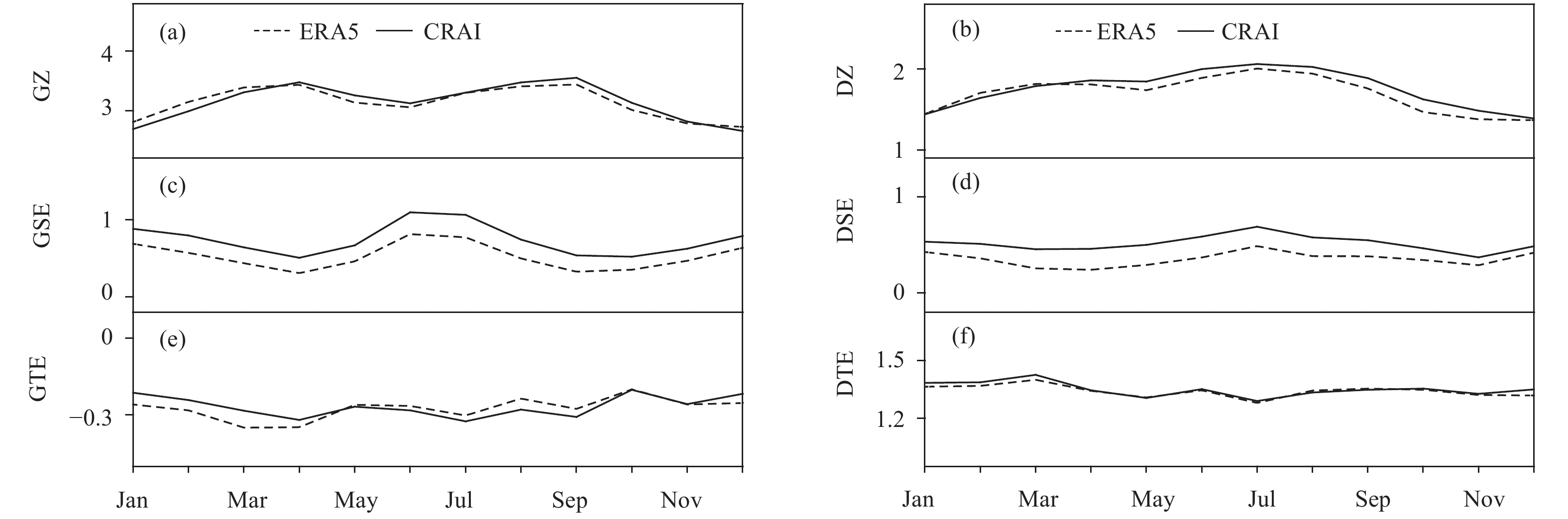Figure 13 Monthly mean (2010–16) time series of the globally averaged (a) GZ, (b) DZ, (c) GSE, (d) DSE, (e) GTE, and (f) DTE from ERA5 (dashed line) and CRAI (solid line). The unit of each variable is W m–2.
4 Conclusions

The global energy cycle diagnosis is an effective way for understanding the general circulation. In this paper, the first Chinese reanalysis datasets (CRAI) produced by the NIMC of CMA is verified in terms of the global atmospheric energy cycle in comparison with the latest version of the ECMWF reanalysis dataset (ERA5) for a 7-yr period (2010–16), based on the overall and monthly analyses.

This study reveals that energy components calculated by these two datasets are highly consistent, while some magnitudes of the reservoir and conversion terms in CRAI are slightly larger. Generally, the discrepancy between the two reanalysis datasets is caused by the conversion in the baroclinic process, especially for CES, which causes the conversion from ASE to KSE. The difference is mainly reflected at low–mid latitudes of the Northern Hemisphere and near the Antarctic below 500 hPa. A further detailed analysis indicates that this discrepancy mainly originates from systematic differences in the temperature and vertical velocity, and is associated with orographic characteristics. It can be concluded that the post-processing of datasets over complex terrains is a possible reason for the discrepancy observed at the regional scale. From monthly analysis, despite the differences in energy reservoir and conversion terms between the two reanalysis datasets, seasonal evolution characteristics are highly consistent. Statistical characteristics play a role in validating the climate change. Although the discrepancy in CES leads to differences in the corresponding generation and dissipation rates, the energy terms associated with the planetary-scale and transient waves do not show differences. Therefore, this discrepancy does not affect the application of CRAI in climate change analysis.

The reanalysis datasets such as ERA5, NCEP-R2, and JRA-25, have been released for climate analysis, and the CRAI dataset will also be available soon. As a preliminary evaluation work, the overall characteristics of the datasets in the globally integrated atmospheric energy cycle are investigated. However, further research on the role of energy transport in the generation and extinction of governing climate systems (e.g., monsoons and tropical cyclones) should be analyzed in detail in future studies.

Appendix

AZ is the zonal available potential energy

 $\hspace{-136pt}{\rm{AZ}} = \dfrac{\gamma }{{2{\rm{g}}}}{([\overline T ] - \{ \overline T \})^2}.$ (A1)

ASE is the stationary eddy available potential energy

 $\hspace{-156pt} {\rm{ASE}} = \dfrac{\gamma }{{2{\rm{g}}}}[{\overline {{T^{*}}} ^2}].$ (A2)

ATE is the transient eddy available potential energy

 $\hspace{-157pt} {\rm{ATE}} = \dfrac{\gamma }{{2{\rm{g}}}}[\overline {{T^{'2}}} ].$ (A3)

KZ is the zonal kinetic energy

 $\hspace{-136pt} {\rm{KZ}} = \dfrac{1}{{2{\rm{g}}}}({[{\overline u} ]^2} + {[{\overline v} ]^2}).$ (A4)

KSE is the stationary eddy kinetic energy

 $\hspace{-135pt} {\rm{KSE}} = \dfrac{1}{{2{\rm{g}}}}[{\overline {{u^{*}}} ^2} + {\overline {{v^{*}}} ^2}].$ (A5)

KTE is the transient eddy kinetic energy

 $\hspace{-137pt} {\rm{KTE}} = \dfrac{1}{{2{\rm{g}}}}[\overline {{u^{'2}}} + \overline {{v^{'2}}} ].$ (A6)

KE is the eddy kinetic energy

 $\hspace{-24pt} \hspace{-126pt} {\rm KE} = {\rm KSE} + {\rm KTE.}$ (A7)

CAS is the conversion from AZ to ASE

 \begin{aligned} \hspace{-48pt}\, {\rm{CAS}} = & - \dfrac{\gamma }{{\rm{g}}}([{\overline v} ^{*}\overline T ^{*}]\dfrac{{\partial [\overline T ]}}{{r\partial \varphi }} + [\overline \omega ^{*}\overline T ^{*}] \\ & \cdot \left(\dfrac{\partial }{{\partial p}}([\overline T ] - \{ \overline T \}) - \dfrac{R}{{p{c_p}}}([\overline T ] - \{ \overline T \}))\right).\end{aligned} \;\;\;\;\;\;\;\;\;\, (A8)

CAT is the conversion from AZ to ATE

 \hspace{-54pt} \begin{aligned}{\rm{CAT}} = & - \dfrac{\gamma }{{\rm{g}}}([\overline {v'{T^{'}}} ]\dfrac{{\partial [\overline T ]}}{{r\partial \varphi }} + [\overline {\omega '{T^{'}}} ] \\ & \cdot \left(\dfrac{\partial }{{\partial p}} ([\overline T ] - \{ \overline T \}) - \dfrac{R}{{p{c_p}}}([\overline T ] - \{ \overline T \}))\right).\end{aligned} \; (A9)

CZ is the conversion from AZ to KZ

 $\hspace{-55pt} {\rm{CZ}} = - \dfrac{R}{{p{\rm{g}}}}([\overline \omega ] - \{ \overline \omega \})([\overline {{T_v}} ] - \{ \overline {{T_v}} \}). \quad\quad\;\;\;\;\,$ (A10)

CES is the conversion from ASE to KSE

 $\hspace{-124pt} {\rm{CES}} = - \dfrac{R}{{p{\rm{g}}}}[\overline \omega ^{*} - \overline {{T_v}} ^{*}]. \;\;\;$ (A11)

CET is the conversion from ATE to KTE

 $\hspace{-140pt}\!{\rm{CET}} = - \dfrac{R}{{p{\rm{g}}}}[\overline {\omega '{T_v}'} ] .$ (A12)

CKS is the conversion from KZ to KSE

 \begin{aligned} {\rm{CKS}} = & -\! \dfrac{1}{{\rm{g}}}([{\overline u} ^{*}{\overline v} ^{*}]\dfrac{{\partial [{\overline u} ]}}{{r\partial \varphi }} \!+\! \dfrac{{t{\rm{g}}\varphi }}{r}[{\overline u} ^{*}{\overline v} ^{*}][{\overline u} ] + [{\overline v} ^{*}{\overline v} ^{*}]\dfrac{{\partial [{\overline v} ]}}{{r\partial \varphi }} \\ & - \dfrac{{t{\rm{g}}\varphi }}{r}[{\overline u} ^{*}{\overline u} ^{*}][{\overline v} ] + [\overline \omega ^{*}{\overline u} ^{*}]\dfrac{{\partial [{\overline u} ]}}{{\partial p}} \\ & + \dfrac{{\partial [{\overline v} ]}}{{\partial p}}[\overline \omega ^{*}{\overline v} ^{*}]). \end{aligned} (A13)

CKT is the conversion from KZ to KSE

 \begin{aligned} \!\!\!\!\! {\rm{CKT}} = & - \dfrac{1}{{\rm{g}}}([\overline {u'v'} ]\dfrac{{\partial [{\overline u} ]}}{{r\partial \varphi }} \\ & + \dfrac{{t{\rm{g}}\varphi }}{r}[\overline {u'v'} ][{\overline u} ] + [\overline {v'v'} ]\dfrac{{\partial [{\overline v} ]}}{{r\partial \varphi }} - \dfrac{{t{\rm{g}}\varphi }}{r}[\overline {u'u'} ][{\overline v} ] \\ & + [\overline {\omega 'u'} ]\dfrac{{\partial [{\overline u} ]}}{{\partial p}} + \dfrac{{\partial [{\overline v} ]}}{{\partial p}}[\overline {\omega 'v'} ]). \end{aligned} (A14)

${C_{{\rm{ATE}} - {\rm{ASE}}}}$ (CATE) is the conversion from ATE to ASE

 \begin{aligned} \!\!\!\!\! {C_{{\rm{ATE}} - {\rm{ASE}}}} = & \frac{\gamma }{{\rm{g}}}\left( {{{\overline {u'T'} }^*}\frac{1}{{r\cos \varphi }}\frac{{\partial {{\overline T}^*}}}{{\partial \lambda }}} \right.\\ & \left. { + \;{{\overline {v'T'} }^*}\frac{1}{{r\cos \varphi }}\frac{{\partial {{\overline T}^*}}}{{r\partial \varphi }}} \right). \quad \quad \quad \quad \quad \quad\;\; \end{aligned} (A15)

${C_{{\rm{KTE}} - {\rm{KSE}}}}$ (CKTE) is the conversion from KTE to KSE

 \! \begin{aligned} {C_{{\rm{KTE}} - {\rm{KSE}}}} = & \frac{1}{{\rm{g}}}\left( {{{\overline {u'u'} }^*}\frac{1}{{r\cos \varphi }}\frac{{\partial {{\bar u}^*}}}{{\partial \lambda }} + {{\overline {u'v'} }^*}\frac{{\partial {{\bar u}^*}}}{{r\partial \varphi }}} \right.\\ & + {\overline {u'v'} ^*}{{\bar u}^*}\frac{{t{\rm{g}}\varphi }}{r} + {\overline {v'v'} ^*}\frac{{\partial {{\bar v}^*}}}{{r\partial \varphi }} - {[\overline {u'u'} ]^*}{{\bar v}^*}\frac{{t{\rm{g}}\varphi }}{r}\\ & \left. { + \;{{\overline {u'v'} }^*}\frac{1}{{r\cos \varphi }}\frac{{\partial {{\bar v}^*}}}{{\partial \lambda }}} \right). \end{aligned} \;\; \;\; \;\; (A16)

GZ, GSE, GTE, DZ, DSE, and DTE are the generation of AZ, ASE, ATE, KZ, KSE, and KTE, respectively.

$Cp$ is the specific heat at constant pressure; g is the gravity of earth; p is the pressure; r is the radius of earth; R is the gas constant; T is the temperature; Tv is the virtual temperature; u is the zonal wind; v is the meridional wind; $\omega$ is the vertical p-velocity; $\varphi$ is the latitude; $\lambda$ is the lontitude; $\gamma = - \dfrac{R}{p}{(\dfrac{\partial }{{\partial p}}[\overline T ] - \dfrac{R}{{Cp}}\dfrac{{[\overline T ]}}{p})^{ - 1}}$ is the stability parameter. ${\overline x}$ is the time mean of x; ${x^{'}}$ is the deviation from time mean; {x} is the global horizontal mean; [x] is the zonal mean; x* is the deviation from zonal mean.

References
 Arpe, K., C. Branković, E. Oriol, et al., 1986: Variability in time and space of energetics from a long series of atmospheric data produced by ECMWF. Contrib. Atmos. Phys., 59, 321–355. Boer, G. J., and S. Lambert, 2008: The energy cycle in atmosphe-ric models. Climate Dyn., 30, 371–390. DOI:10.1007/s00382-007-0303-4 Dee, D. P., S. M. Uppala, A. J. Simmons, et al., 2011: The ERA-Interim reanalysis: Configuration and performance of the data assimilation system. Quart. J. Roy. Meteor. Soc., 137, 553–597. DOI:10.1002/qj.828 Dickinson, R. E., 1969: Theory of planetary wave-zonal flow interaction. J. Atmos. Sci., 26, 73–81. DOI:10.1175/1520-0469(1969)026<0073:TOPWZF>2.0.CO;2 Gao, H., L. X. Chen, J. H. He, et al., 2006: Characteristics of zo-nal propagation of atmospheric kinetic energy at equatorial region in Asia. Acta Meteor. Sinica, 20, 86–94. Kalnay, E., M. Kanamitsu, R. Kistler, et al., 1996: The NCEP/NCAR 40-year reanalysis project. Bull. Amer. Meteor. Soc., 77, 437–472. DOI:10.1175/1520-0477(1996)077<0437:TNYRP>2.0.CO;2 Kanamitsu, M., W. Ebisuzaki, J. Woollen, et al., 2002: NCEP–DOE AMIP-II Reanalysis (R-2). Bull. Amer. Meteor. Soc., 83, 1631–1644. DOI:10.1175/BAMS-83-11-1631 Kim, Y. H., and M. K. Kim, 2013: Examination of the global Lorenz energy cycle using MERRA and NCEP-reanalysis 2. Climate Dyn., 40, 1499–1513. DOI:10.1007/s00382-012-1358-4 Kistler, R., E. Kalnay, W. Collins, et al., 2001: The NCEP–NCAR 50-year reanalysis: Monthly means CD-ROM and documentation. Bull. Amer. Meteor. Soc., 82, 247–268. DOI:10.1175/1520-0477(2001)082<0247:TNNYRM>2.3.CO;2 Li, L. M., A. P. Ingersoll, X. Jiang, et al., 2007: Lorenz energy cycle of the global atmosphere based on reanalysis datasets. Geophys. Res. Lett., 34, 16813. DOI:10.1029/2007GL029985 Li, Q. Q., and Q. G. Zhu, 1995: Analysis on the source and sink of kinetic energy of atmospheric 30–60 day period oscillation and the probable causes. Acta Meteor. Sinica, 9, 420–431. Lorenz, E. N., 1955: Available potential energy and the maintenance of the general circulation. Tellus, 7, 157–167. DOI:10.3402/tellusa.v7i2.8796 Luo, Z. X., 1994: Effect of energy dispersion on the structure and motion of tropical cyclone. Acta Meteor. Sinica, 8, 51–59. Marques, C. A. F., A. Rocha, J. Corte-Real, et al., 2009: Global atmospheric energetics from NCEP-reanalysis 2 and ECMWF-ERA40 reanalysis. Int. J. Climatol., 29, 159–174. DOI:10.1002/joc.1704 Marques, C. A. F., A. Rocha, and J. Corte-Real, 2010: Comparative energetics of ERA-40, JRA-25 and NCEP-R2 reanalysis, in the wave number domain. Dyn. Atmos. Oceans, 50, 375–399. DOI:10.1016/j.dynatmoce.2010.03.003 Onogi, K., H. Koide, M. Sakamoto, et al., 2005: JRA-25: Japanese 25-year re-analysis project—progress and status. Quart. J. Roy. Meteor. Soc., 131, 3259–3268. DOI:10.1256/qj.05.88 Oort, A. H., 1964: On estimates of the atmospheric energy cycle. Mon. Wea. Rev., 92, 483–493. DOI:10.1175/1520-0493(1964)092<0483:OEOTAE>2.3.CO;2 Oort, A. H., and J. P. Peixóto, 1974: The annual cycle of the energetics of the atmosphere on a planetary scale. J. Geophys. Res., 79, 2705–2719. DOI:10.1029/JC079i018p02705 Rienecker, M. M., M. J. Suarez, R. Gelaro, et al., 2011: MERRA: NASA’s modern-era retrospective analysis for research and applications. J. Climate, 24, 3624–3648. DOI:10.1175/JCLI-D-11-00015.1 Simmons, A. J., and B. J. Hoskins, 1980: Barotropic influences on the growth and decay of nonlinear baroclinic waves. J. Atmos. Sci., 37, 1679–1684. DOI:10.1175/1520-0469(1980)037<1679:BIOTGA>2.0.CO;2 Steinheimer, M., M. Hantel, and P. Bechtold, 2008: Convection in Lorenz’s global energy cycle with the ECMWF model. Tellus A, 60, 1001–1022. DOI:10.1111/j.1600-0870.2008.00348.x Stone, P. H., 1978: Baroclinic adjustment. J. Atmos. Sci., 35, 561–571. DOI:10.1175/1520-0469(1978)035<0561:BA>2.0.CO;2 Ulbrich, U., and P. Speth, 1991: The global energy cycle of stationary and transient atmospheric waves: Results from ECMWF analyses. Meteor. Atmos. Phys., 45, 125–138. DOI:10.1007/BF01029650 Uppala, S. M., P. W. KÅllberg, A. J. Simmons, et al., 2005: The ERA-40 re-analysis. Quart. J. Roy. Meteor. Soc., 131, 2961–3012. DOI:10.1256/qj.04.176 von Storch, J. S., C. Eden, I. Fast, et al., 2012: An estimate of the Lorenz energy cycle for the world ocean based on the STORM/NCEP simulation. J. Phys. Oceanogr., 42, 2185–2205. DOI:10.1175/JPO-D-12-079.1 Zhao, B., and B. Zhang, 2014: Diagnostic study of global energy cycle of the GRAPES global model in the mixed space–time domain. J. Meteor. Res., 28, 592–606. DOI:10.1007/s13351-014-3072-0